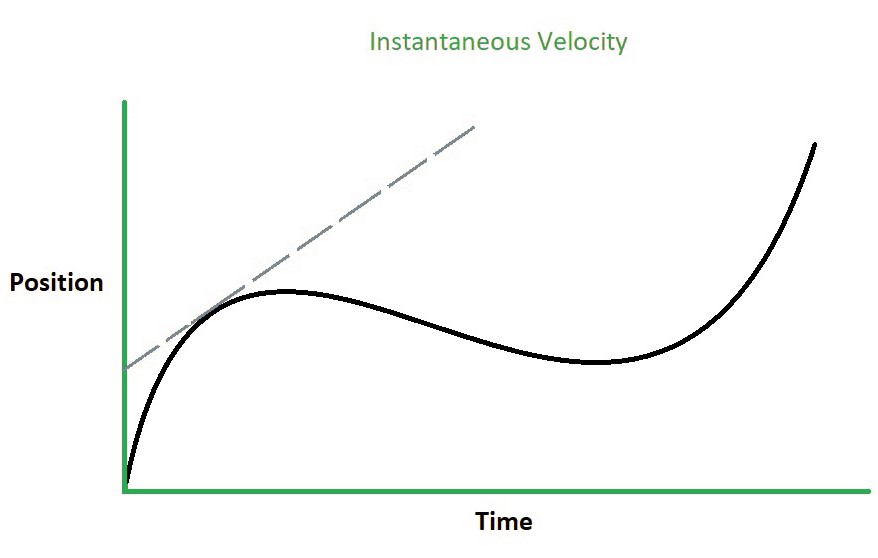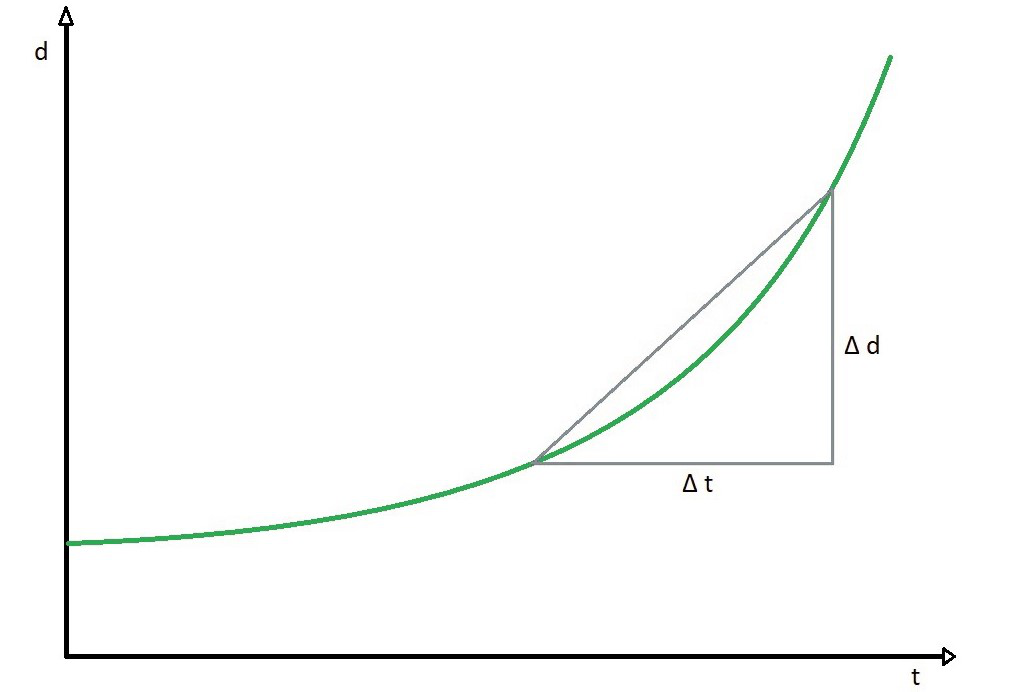# How does Instantaneous Velocity differ from Average Velocity?

• Last Updated : 16 May, 2022

Velocity is a crucial topic in physics. Many qualities of a body, such as kinetic energy and viscosity, are influenced by its velocity. The term velocity describes how quickly or slowly an object is moving. Velocity can be defined as the rate of change of the object’s position with respect to time and frame of reference. In disciplines as diverse as kinematics, kinetics, dynamics, astrophysics, and engineering, the idea of velocity is usually applied. To excel in such disciplines, it is critical to have a thorough knowledge of the notions of instantaneous velocity and average velocity. In this article, we’ll look closely at how Instantaneous Velocity differs from Average Velocity.

### Instantaneous Velocity

The rate of change of position over a relatively short time span is called instantaneous velocity or the velocity of an object at that instant of time. The instantaneous velocity may be calculated by multiplying the object’s instantaneous speed by the direction in which it is traveling at the time. The rate of change of displacement is described as velocity in Classical physics. The terms “velocity” refer to vector quantity. They have a numerical value as well as a direction. An object with uniform velocity may have the same immediate velocity as its standard velocity. The International System of Units (SI) of instantaneous velocity is meters per second (m/s).

It’s calculated as the average velocity divided by the minimum period. The ratio of total displacement to total time can be used to compute average velocity. The displacement is proportional to the time interval. The limit of this ratio between time and displacement is known as instantaneous velocity.Where

• V(t) is Instantaneous velocity at time t
• x denotes displacement
• t denotes time
• Δt is the small-time interval

The slope of the tangent line to the function at that place is equal to the instantaneous velocity at any given point on a function x (t). The slope of the distance-time graph, often known as the x-t graph, can also be used to illustrate it. The instantaneous velocity formula is:The velocity between two positions in a time limit where the time between them progressively becomes 0 is called instantaneous velocity. The location of x in relation to the function of t is denoted by X(t). The following is the equation for the average velocity between the two points:Let, t1 and t2 andThe Δt must be 0 in order to determine instantaneous velocity,

Usingas the limit and putting the expressions into the equationThus, V(t) = dx/dt

### Average Velocity

The average velocity is calculated by dividing the change in total displacement by the total time taken. The average velocity of an item is always less than or equal to its average speed. This may be demonstrated by noting that, whereas distance is always strictly rising, displacement can change direction as well as increase or decrease in magnitude. The International System of Units (S.I) average velocity is meters per second (m/s)Where,

• x1 is the final displacement
• x0 is the initial displacement
• t1 is the time taken at final position
• t0 is the time taken at initial position

The average velocity is defined as the slope of a secant line connecting two locations with t coordinates corresponding to the time period’s borders.The average velocity is the same as the velocity averaged over time, or its time-weighted average, which may be determined as the velocity’s time integral.Where we can find out,And,

△t = t1 − t0

Difference between Instantaneous Velocity and Average Velocity

### Sample Questions

Question 1: When the position of the provided particle is x(t) = 2.0t + 0.73m, calculate the instantaneous velocity at t = 3.0s.

Solution:

Given

x(t) = 2.0t + 0.73

t = 3 secv = 2t + 3 × 0.7 × t2

v = 2t + 2.1t2

At t = 3sec

v = 2 × 3 + 3 × 0.7 × 32

v = 6 + 2.1 × 9

v = 6 + 18 – 9

v = 24.9 m/s

Question 2: Calculate the instantaneous velocity at the time (t) = 4 sec using the function x = 3t2 – 5t + 2 to get the position of a moving bus.

Solution:

Given,

x = 3t2 – 5t + 2

t = 4secV = 6t – 5

At t = 4 sec

V = 6t – 5

V = 6 × 4 – 5

V = 19 m/s

Question 3: What is the shortest time a predator will take to catch its prey at a distance of 200m if its average velocity is 90 km/hr?

Solution:

Given,V = 90 × 5/18 m/sec

V = 25m/seca = 625/400

a = 1.56 m/st2 = 400/1.56

t2 = 256.4

t2 = 16 sec

Question 4: S(t) = 9t + 12t2  is the equation of motion for a car traveling in a straight path for 15 seconds before crashing. Calculate the instantaneous velocity at the 9th-second interval.

Solution:

Given,

S(t) = 9t + 12t2V = 9 + 24t

Therefore Vinst at t = 9

V = 9 + 24(9)

V = 225 m/s

Question 5: With a function, x = 7t2+ 3t + 3a given projectile moves in a straight line for time (t) = 2s. Calculate the instantaneous velocity of a moving object.

Solution:

Given,

x = 7t2 + 3t + 3

t = 2 secVinst = 14t + 3

At t = 2 sec

V(2) = 14(2) + 3

V(2) = 31m/s

Question 6: In 4 minutes, the bus driver travels 15 kilometers down the road. He then took a step back and drove 9 kilometers down the road in 2 minutes. What is his typical speed?

Solution:Average Velocity (vavg) =Average Velocity (vavg) = 6/6

Average Velocity (vavg) =1 km/m

Question 7: Calculate a person’s average velocity at a specific time when he walks 5 meters in 3 seconds and 15 meters in 5 seconds in a straight line along the x-axis.

Solution:

Given,

The initial distance traveled xi = 5 m,

Final distance traveled, xf = 15m,

Initial time interval = 3 s,

Final time interval tf = 5 s,Average Velocity (vavg) = 5 m/s.

My Personal Notes arrow_drop_up